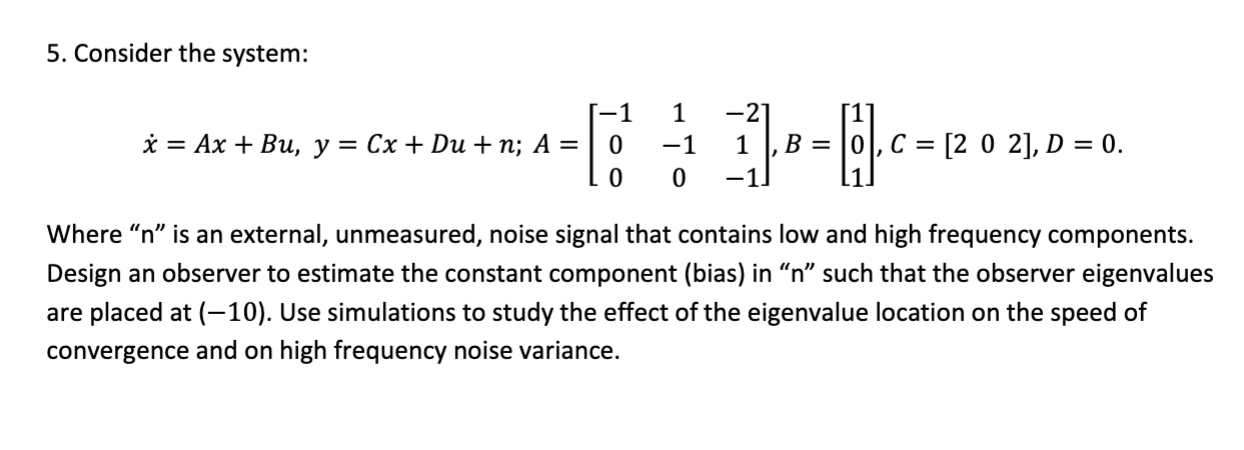# Question 5. Consider the system: $\dot{x}=A x+B u, y=C x+D u+n ; A=\left[\begin{array}{ccc} -1 & 1 & -2 \\ 0 & -1 & 1 \\ 0 & 0 & -1 \end{array}\right], B=\left[\begin{array}{l} 1 \\ 0 \\ 1 \end{array}\right], C=\left[\begin{array}{lll} 2 & 0 & 2 \end{array}\right], D=0 .$ Where " $$n$$ " is an external, unmeasured, noise signal that contains low and high 5. Consider the system: $\dot{x}=A x+B u, y=C x+D u+n ; A=\left[\begin{array}{ccc} -1 & 1 & -2 \\ 0 & -1 & 1 \\ 0 & 0 & -1 \end{array}\right], B=\left[\begin{array}{l} 1 \\ 0 \\ 1 \end{array}\right], C=\left[\begin{array}{lll} 2 & 0 & 2 \end{array}\right], D=0 .$ Where " $$n$$ " is an external, unmeasured, noise signal that contains low and high frequency components. Design an observer to estimate the constant component (bias) in " $$n$$ " such that the observer eigenvalues are placed at (-10). Use simulations to study the effect of the eigenvalue location on the speed of convergence and on high frequency noise variance.YXVBWH The Asker · Electrical EngineeringTranscribed Image Text: 5. Consider the system: $\dot{x}=A x+B u, y=C x+D u+n ; A=\left[\begin{array}{ccc} -1 & 1 & -2 \\ 0 & -1 & 1 \\ 0 & 0 & -1 \end{array}\right], B=\left[\begin{array}{l} 1 \\ 0 \\ 1 \end{array}\right], C=\left[\begin{array}{lll} 2 & 0 & 2 \end{array}\right], D=0 .$ Where " $$n$$ " is an external, unmeasured, noise signal that contains low and high frequency components. Design an observer to estimate the constant component (bias) in " $$n$$ " such that the observer eigenvalues are placed at (-10). Use simulations to study the effect of the eigenvalue location on the speed of convergence and on high frequency noise variance.
More
Transcribed Image Text: 5. Consider the system: $\dot{x}=A x+B u, y=C x+D u+n ; A=\left[\begin{array}{ccc} -1 & 1 & -2 \\ 0 & -1 & 1 \\ 0 & 0 & -1 \end{array}\right], B=\left[\begin{array}{l} 1 \\ 0 \\ 1 \end{array}\right], C=\left[\begin{array}{lll} 2 & 0 & 2 \end{array}\right], D=0 .$ Where " $$n$$ " is an external, unmeasured, noise signal that contains low and high frequency components. Design an observer to estimate the constant component (bias) in " $$n$$ " such that the observer eigenvalues are placed at (-10). Use simulations to study the effect of the eigenvalue location on the speed of convergence and on high frequency noise variance.